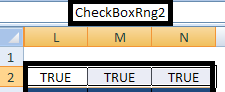# Excel : Interactive Chart Using Check Boxes

This tutorial explains how to create interactive chart with check boxes in Excel.

Check Box

The Check Box form Control allows the selection of multiple items from a list of items. When one item is selected, the other check box can also be checked.Interactive Chart with Check Boxes
Important Steps

2. Add Group Box and group all the check boxes (Use CTRL SHIFT to select check boxes)

3. Define Name Range for cell reference of 3 check boxes combined (Except "All" box).Name Range

4. Press ALT F11 and Go to Insert >> Module and paste the following code
Option Explicit
Sub ChartUsingCheckBox()
Dim rCell As Range
Range("O2").Formula = "FALSE"
For Each rCell In ActiveSheet.Range("CheckBoxRng2").Cells
rCell.EntireColumn.Hidden = Not rCell.Value
Next
If Range("L2").Value = True And Range("M2").Value = True And Range("N2").Value = True Then
Range("O2").Formula = "TRUE"
ElseIf Range("L2").Value = False And Range("M2").Value = False And Range("N2").Value = False Then
Range("O2").Formula = "FALSE"
End If
End Sub
Sub ChartUsingCheckBox2()
If Sheet1.Range("O2").Value = True Then
Range("L2:N2").Formula = "TRUE"
Columns("L:N").EntireColumn.Hidden = False
ElseIf Sheet1.Range("O2").Value = False Then
Range("L2:N2").Formula = "FALSE"
Columns("L:N").EntireColumn.Hidden = True
End If
End Sub
Note :
O2 - Cell link of "All" CheckBox
L2,M2,N2 - Cell link of the remaining 3 check boxes
5. Assign the "ChartUsingCheckBox2" macro to "All" checkbox and "ChartUsingCheckBox" to each of the remaining 3 check boxes.Share
Related Posts1.